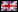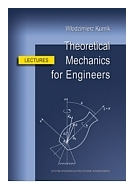wprowadź własne kryteria wyszukiwania książek: (jak szukać?) książki polskie książki zagraniczne polskie i zagraniczne tytuł autor wydawca opis wszędzie
 Twój koszyk:   0 zł   zamówienie wysyłkowe >>>
Strona główna > opis książkiEnglish version
Książki:

Zamów informacje o nowościach z wybranego tematu

Informacje:

Regulamin zakupów

Szukasz książki?
Pomożemy Ci!
512 994 090

Napisz
poczta@ksiazki24h.pl# KURNIK W.

wydawnictwo: WYD PW , rok wydania 2017, wydanie I

cena netto: 59.40 Twoja cena  56,43 zł + 5% vat - dodaj do koszyka

### Lectures

This book is a written aid to the lectures on Theoretical Mechanics, being given to the international students of Mechanical Engineering at the Faculty of Automotive and Construction Machinery Engineering of the Warsaw University of Technology. The main objective of the book is to provide the undergraduate students with the necessary knowledge and skills to solve mechanical problems that can occur in their engineering job, or earlier – during studies – within semester or diplome projects.

PREFACE  11

PART I. THEORETICAL MECHANICS I . 15

Chapter 1: Introduction 17
Lecture 1
1.1. What is Mechanics? . 17
1.2. Classifi cation of Mechanics . 17
1.3. Historical outline  19
1.4. Mechanics as a theory  20
1.5. Parts of Mechanics . 22
1.6. Mechanical quantities and system of physical units  22
1.7. Useful knowledge preceding Mechanics . 23
Review questions to Chapter 1  24

Chapter 2: Vectors and vector calculus  25
Lecture 2
2.1. Scalars and vectors in Mechanics  25
2.2. Geometric and analytic description of vectors . 25
2.3. Vector calculus . 26
2.4. Vector functions of time . 29
Review questions to Chapter 2  34

Chapter 3: Geometry of masses  35
Lecture 3
3.1. What is geometry of masses? . 35
3.2. First moments and center of mass of a multi-particle system and a body . 36
3.3. Calculation of fi rst moments and positions of mass centers of bodies  38
3.4. Pappus-Guldinus rules . 46
Lecture 4
3.5. Moments and products of inertia (second moments)  49
3.6. Calculation of second moments . 53
3.7. Transformation of the second moments due to translation of reference frame 58
Lecture 5
3.8. Transformation of second moments due to rotation of the reference frame  64
3.9. Principal axes and principal moments of inertia  68
3.10. Ellipsoid of inertia of a body at a point  73
Review questions to Chapter 3  76

Chapter 4: Statics of mechanical systems  77
Lecture 6
4.1. Subject, problems and methods of statics  77
4.2. Classifi cation of forces (and free moments) in statics . 80
4.3. Constraints and supports of mechanical systems . 82
4.4. Equivalent reduction of forces and moments acting on a body . 84
4.5. Geometric conditions of equilibrium of mechanical systems  92
Lecture 7
4.6. Friction in statics of mechanical systems . 96
4.7. Friction-induced zones of equilibrium and intervals of loads  98
4.8. Friction-induced static indeterminability . 101
4.9. Duality of loss of equilibrium  102
4.10. Self-locking and jamming effects  108
Lecture 8
4.11. Rolling resistance . 114
4.12. Belt friction  117
4.13. Plane trusses . 119
Review questions to Chapter 4  125

Chapter 5: Kinematics of a particle . 127
Lecture 9
5.1. Geometric and analytic description of position of a particle . 127
5.2. Path of a particle in space . 129
5.3. Velocity and acceleration of a particle . 135
5.4. Components of velocity and acceleration in the cylindrical reference frame  137
Lecture 10
5.5. Components of velocity and acceleration in natural directions . 141
5.6. Rectilinear motion of a particle  144
5.7. Curvilinear motion of a particle in a uniform fi eld of acceleration  149
5.8. Motion of a particle in a central fi eld of acceleration  150
Review questions to Chapter 5  152

Chapter 6: Dynamics of a particle  153
Lecture 11
6.1. Introduction to dynamics  153
6.2. Dynamics of a free particle  154
6.3. Motion of a particle under force dependent on position  155
6.4. Motion of a particle under force dependent on velocity  162
Lecture 12
6.5. Motion of a particle under the Lorentz force . 167
6.6. Motion of a particle under force dependent on time  169
6.7. Dynamics of a constrained particle  171
6.8. Linear momentum law of a particle . 175
6.9. Angular momentum law of a particle . 179
Lecture 13
6.10. Work and power of a force acting on a particle . 183
6.11. Kinetic energy of a particle and kinetic energy law . 186
6.12. Kinetic energy law in a potential fi eld of force  188
Review questions to Chapter 6  194

Chapter 7: Dynamics of multi-particle systems  195
Lecture 14
7.1. Defi nition and basic properties of a multi-particle system . 195
7.2. Equations of motion of a multi-particle system . 198
7.3. Linear momentum law of a multi-particle system . 203
7.4. Law of motion of the mass center of a multi-particle system  205
Lecture 15
7.5. Angular momentum law of a system of particles . 210
7.6. Kinetic energy law of a system of particles . 213
7.7. Kinetic energy law in case of potential forces . 215
Review questions to Chapter 7  222

PART II. THEORETICAL MECHANICS II 223

Chapter 8: Kinematics of a rigid body . 225
Lecture 1
8.1. Description of the position of a body in space . 225
8.2. Classifi cation of motions of a rigid body . 231
8.3. Velocity of points of a body in arbitrary motion  233
Lecture 2
8.4. Acceleration of points of a body in arbitrary motion . 236
8.5. Velocity and acceleration of points of a body in translatory motion  240
8.6. Velocity and acceleration of points of a body in rotation about a fi xed point   242
Lecture 3
8.7. Velocity and acceleration of points of a body in plane motion  248
8.8. Velocity and acceleration of points of a body in screw motion . 256
Review questions to Chapter 8  258

Chapter 9: Resultant motion of a particle . 259
Lecture 4
9.1. Description of motion of a particle in different reference frames  259
9.2. Velocity and acceleration of a particle in resultant motion  262
Lecture 5
9.3. Dynamics of a particle in relative motion  269
Review questions to Chapter 9  280

Chapter 10: Dynamics of a rigid body . 281
Lecture 6
10.1. Kinetic energy of a rigid body  281
10.2. Kinetic energy law of a rigid body . 286
Lecture 7
10.3. Linear momentum of a body and linear momentum law . 291
10.4. Angular momentum of a body and angular momentum law  294
10.5. Equations of motion of a body resulting from linear and angular momentum laws   300
10.6. Dynamics of a body in translatory motion . 301
Lecture 8
10.7. Dynamics of rotation of a body about a fi xed axis . 304
10.8. Reactions in bearings of a body rotating about a fi xed axis . 307
Lecture 9
10.9. Dynamics of a body in rotation about a fi xed point . 316
10.10. Gyroscopic phenomenon  322
10.11. Dynamics of a body in plane motion  324
Lecture 10
10.12. Dynamics of a rolling wheel  327
10.13. Dynamics of vehicles . 331
Review questions to Chapter 10  336

Chapter 11: Elements of analytical mechanics 337
Lecture 11
11.1. Motivation for studies of analytical mechanics  337
11.2. Constraints and generalized coordinates of a multi-particle system  338
11.3. Virtual displacements in a multi-particle system . 341
11.4. Principle of virtual work . 343
11.5. Principle of virtual work in case of a rigid body  349
Lecture 12
11.6. D’Alembert’s principle . 351
11.7. Lagrange’s equations  355
Review questions to Chapter 11 . 366

Chapter 12: Elementary impact theory . 367
Lecture 13
12.1. Impact forces  367
12.2. Dynamics of a particle under impact force  368
12.3. Collision of a particle with a resting body . 370
12.4. Collision of two particles . 374
12.5. Effect of impact on a rigid body . 377
Lecture 14
12.6. Effect of impact on a body rotating about a fi xed axis . 380
12.7. Collision of two bodies in plane motion  385
Review questions to Chapter 12  392

Chapter 13: Dynamics of a particle of continuously varying mass . 393
Lecture 15
13.1. Equation of motion of a particle of variable mass . 393
13.2. Dynamics of a rotating body with a variable moment of inertia  399
13.3. Equation of motion of a rocket . 401
Review questions to Chapter 13  404

REFERENCES  405

406 pages, Paperback

Po otrzymaniu zamówienia poinformujemy,
czy wybrany tytuł polskojęzyczny lub anglojęzyczny jest aktualnie na półce księgarni.